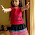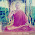## Saturday, March 13, 2010

Lecture Slides

### Lesson 1 Lesson 2 Lesson 3 Lesson 4 Lesson 5 Lesson 6 Lesson 7 Lesson 8 Lesson 9 Lesson 10 Lesson 11 Lesson 12 Lesson 13 Lesson 14 Lesson 15 Lesson 16 Lesson 17 Lesson 18 Lesson 19 Lesson 20 Lesson 21 Lesson 22 Lesson 23 Lesson 24 Lesson 25 Lesson 26 Lesson 27 Lesson 28 Lesson 29 Lesson 30 Lesson 31 Lesson 32 Lesson 33 Lesson 34 Lesson 35 Lesson 36 Lesson 37 Lesson 38 Lesson 39 Lesson 40

1.2.3.thanks allots

4.thank you so much for the help!!

5.thank u............

6.7.Thank U:)
frm Raj Salunke, gokak

8.excellent material,thank u so much

9.Thanks for guiding.

10.Portable air conditioner reviews.
All you need to choose the best portable air conditioner.

air conditionin
air conditioner
ac air conditioning
furnace
hvac